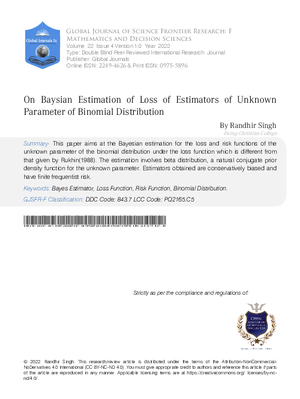### On Baysian Estimation of Loss of Estimators of Unknown Parameter of Binomial Distribution

Authors

• Randhir Singh

Keywords:

Bayes Estimator, Loss Function, Risk Function, Binomial Distribution

Abstract

This paper aims at the Bayesian estimation for the loss and risk functions of the unknown parameter of the binomial distribution under the loss function which is different from that given by Rukhin 1988 The estimation involves beta distribution a natural conjugate prior density function for the unknown parameter Estimators obtained are conservatively biased and have finite frequentist risk

How to Cite

Randhir Singh. (2022). On Baysian Estimation of Loss of Estimators of Unknown Parameter of Binomial Distribution. Global Journal of Computer Science and Technology, 22(F4), 37–40. Retrieved from https://computerresearch.org/index.php/computer/article/view/102247Published

2022-11-01

Issue

Section

Articles